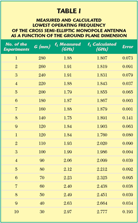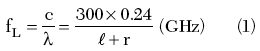In this article, a novel design for a finite ground plane cross-shaped semi-elliptic monopole antenna is presented and investigated. The bandwidth of the proposed antenna can cover a number of wireless communications. By cutting four narrow slits into the lobes of the cross-shaped monopole antenna, a band-reject from 5.25 to 6.05 GHz is obtained.

Characteristics of the Cross Semi-elliptic Monopole Antenna

Figure 1 shows the geometry of the cross semi-elliptic monopole antenna. The monopole is made of 0.1 mm thick copper sheets, which are located vertically above a square ground plane of dimension GG mm2. The cross semi-elliptic monopole is made by using two semi-elliptic conducting plates. The length of the major axis is 2 H1; the length of the minor axis is W1. A photograph of the fabricated prototype antenna is shown in Figure 2. The measured return loss of the proposed antenna is plotted in Figure 3, where the antenna dimensions are H1 = 28 mm, H = 0.5 mm, W1 = 32 mm and G = 120 mm. It shows that a very large impedance bandwidth, from 1.85 to more than 12 GHz, can be obtained with this design. It can cover the UWB and future wireless applications above 10 GHz. Please note that since the SMA connector offers good performance from only DC to 12 GHz, the measurement frequency is up to 12 GHz. When an operating frequency greater than 12 GHz is desired, the use of a high performance SMA or other high frequency connector is suggested. In addition, for most modern portable devices, the size of the overall antenna must be kept small. In a monopole antenna, reducing the size of the monopole and the ground is an important issue. In the conducted experiments, the height of the cross-elliptic monopole antenna, corresponding to 0.255?, is larger than that of the cross semi-elliptic monopole antenna, corresponding to 0.172? for the same size ground plane. Therefore, the use of the cross semi-elliptic monopole antenna has the advantage of a compact size compared to the cross-elliptic monopole antenna.The design parameters of the proposed antenna can easily be determined. When the size of the ground plane of the antenna is much larger than that of the antenna itself, the lowest operating frequency fL is determined from the following formulawhere

r = equivalent radius9 of the monopole antenna, determined bywhere

A = area of the proposed antenna= total height of the proposed antenna

In most antenna designs, the dimensions of the ground plane have a strong effect on the lowest operating frequency. This was observed and studied. The conventional monopole antenna usually has a large ground plane,8,9 greater than ?/2 × ?/2, to fit the image theory. When the dimensions of the ground plane are dramatically reduced, the lowest operating frequency is increased. The lowest operating frequency of the cross semi-elliptic monopole antenna as a function of the ground plane dimensions was measured and listed in Table 1. The other antenna dimensions are the same as in Figure 3. It can be seen that the lowest operating frequency is increasing when the side length G of the ground plane is less than that 120 mm, corresponding to a half wavelength. Consequently, the variance of the lowest operating frequency must be considered when the side length is smaller than ?/2.From Table 1, a formula can be found by fitting the data using the curve-fitting technique of MATLAB (using the polynomial y = a + bx). Here, the height of the probe feed is H = 0.5 mm, and the lengths of the major axis and of the minor axis are fixed. When the side length of the ground plane is smaller than ?/2, the lowest operating frequency can be obtained from

FL = 3.1160 – 0.0113 G

where

FL = the lowest operating frequency in GHz
G = the side length of the ground plane in mm

Similarly, when the side length of the ground plane is larger than ?/2, the lowest operating frequency can be obtained from

FL = 1.975 – 0.006 G

A more accurate formula could be obtained by using a higher order polynomial from MATLAB but, as can be seen, the measured and curve-fitting results of the lowest operating frequency are in good agreement. The antenna bandwidth is slightly reduced when the side length G of the ground plane is reduced, but the impedance of the proposed antenna still has a very large bandwidth of approximately 10 GHz. Figure 4 shows the measured radiation patterns in the y-z plane at f = 2.45, 4, 5, 6, 8 and 10 GHz for the proposed antenna. The x-y plane radiation patterns are almost omni-directional and are not shown for brevity. It is also noted that the patterns of the proposed antenna are dipole-like radiation, which makes the proposed antenna suitable for practical UWB applications.Band-rejected Cross Semi-elliptic Monopole Antenna

The configuration of the band-rejected cross semi-elliptic monopole antenna is shown in Figure 5. The four narrow slits of length H2 and width W2 are symmetrically cut into the cross semi-elliptic monopole antenna. They strongly disturb the current distribution in the specified band-rejected frequency. It leads to the band-reject characteristics of the proposed antenna. The length of the narrow slit is approximately 0.179?c, where ?c is the center frequency of the rejected band. A photograph of the band-rejected proposed antenna is shown in Figure 6. Figure 7 shows the measured and simulated return losses of the band-rejected proposed antenna with operating bandwidths of 1.85 to 5.24 GHz and 6.04 to 12 GHz with a return loss greater than 10 dB. The antenna dimensions were H1 = 28 mm, W1 = 32 mm, H = 0.5 mm, W2 = 2 mm, W3 = 16 mm, H2 = 9.5 mm and G = 120 mm. The impedance bandwidth obtained with the present design fits the requirement of the proposed DS-UWB.11 The lowest operating frequency with finite ground dimensions of the band-rejected proposed antenna can be determined by using the formulas of the cross semi-elliptic monopole antenna given previously. By observing the influence of various parameters on the antenna performance, it was found that the position and width of the narrow slits slightly affect the band-rejected properties. The dominant factors in the proposed antenna design are the length of the narrow slits cut into the cross semi-elliptic monopole antenna.

Figure 8 compares the real and imaginary impedances of the cross semi-elliptic monopole antenna and the band-rejected cross semi-elliptic monopole antenna. It is observed that the real impedance of the proposed band-rejected antenna is dramatically increased in the band-rejected frequency band and the imaginary impedance of the proposed band-rejected antenna is dramatically changing in the rejected frequency band, which leads to the band-rejected characteristics of the cross semi-elliptic monopole antenna with four narrow slits. The increased real part of the impedance within the rejected frequency band of the proposed antenna is contrary to that of the band-notch monopole antenna.10

Figure 9 shows the measured radiation patterns in the y-z plane at f = 2.45, 4, 5, 7, 9 and 10 GHz for the proposed band-rejected antenna. The x-y plane radiation patterns are almost omni-directional and are not shown for brevity. It is also noted that the patterns of the proposed antenna are dipole-like radiation, which makes the proposed antenna suitable in practical UWB applications. The measured x-y plane peak antenna gain for the proposed antenna and the band-rejected proposed antenna over the impedance bandwidth is shown in Figure 10. It is obvious that, in the proposed antenna with four narrow slits, the x-y plane peak gain is significantly affected in the band-rejected bandwidth. The measured y-z plane peak antenna gain for the proposed antenna and the band-rejected proposed antenna over the impedance bandwidth is also presented in Figure 11. The proposed antenna shows a peak antenna gain of about 6.88 dBi, with a gain variation less than 3.6 dB. The band-rejected proposed antenna shows a peak antenna gain of about 6.80 dBi and a gain variation less than 3.6 dB.

Conclusion

A cross semi-elliptic monopole antenna and a band-rejected cross semi-elliptic monopole antenna, with finite ground plane, have been implemented and investigated. Experimental results show that the impedance bandwidths obtained from the two designs of antenna fit the requirements for UWB applications. The dipole-like radiation patterns over the operating bandwidth are also shown in this article. The proposed antennas have a compact size, compared to the corresponding elliptic monopole antenna. The proposed design of this antenna with finite ground is suitable for practical UWB applications.

Acknowledgments

The authors gratefully acknowledge the support of this study by the National Science Council, Taiwan, Republic of China, under contract number NSC94-2213-E-218-006.

References

1. FCC, First Report and Order, FCC 02-48, February 14, 2002.

2. D. Porocino, “Ultrawideband Radio Technology: Potential and Challenges Ahead,” IEEE Communications Magazine, 2003, pp. 66–74.

3. G.R. Aiello and G.D. Rogerson, “Ultrawideband Wireless Systems,” IEEE Microwave Magazine, June 2003, pp. 36–47.

4. H. Schantz, The Art and Science of Ultrawideband Antenna, Artech House, Norwood, MA, 2005.

5. M.J. Ammann, “Improve Pattern Stability for Monopole Antennas with Ultrawideband Impedance Characteristics,” 2003 IEEE Antennas and Propagation International Symposium Digest, Vol. 2, pp. 818–821.

6. Y. Rikuta and R. Kohno, “Characteristics of Planar and Orthogonal Planar Monopole Antenna with Dual Frequency for UWB System,” 2004 IEEE Antennas and Propagation International Symposium Digest, Vol. 1, pp. 171–175.

7. P.V. Anob, K.P. Ray and G. Kumar, “Wideband Orthogonal Square Monopole Antennas with Semi-circular Base,” 2001 IEEE Antennas and Propagation International Symposium Digest, Vol. 1, pp. 294–297.

8. C.A. Balanis, Antenna Theory: Analysis and Design, Second Edition, John Wiley & Sons Inc., Hoboken, NJ, 1997.

9. N.P. Agarwall, G. Kumar and K.P. Ray, “Wide Band Monopole Antenna,” IEEE Transactions on Antennas and Propagation, Vol. 46, 1998, pp. 294–295.

10. S.W. Su, K.L. Wong and C.L. Tang, “Band-notched Ultrawideband Planar-monopole Antenna,” Microwave and Optical Technology Letters, Vol. 44, February 2005, pp. 217–219.

11. R. Fisher, R. Kohno, H. Ogawa, H. Zhang, M. McLaughlin and M. Welborn, DS-UWB Proposal Update, Doc.: IEEE 802.15-04/140r3, May 2004.

Wen-Shan Chen received his BS degree in electronic engineering technology from the Taiwan Institute of Technology (now the National Taiwan University of Technology) and his PhD degree from Sun Yat-Sen University, Kaohsiung, Taiwan, ROC, in 2001. From 2001 to 2002, he was an assistant professor at the Chien-Kuo Institute of Technology, Changhua, Taiwan, ROC. He is currently an assistant professor of electrical engineering at the Southern Taiwan University of Technology, Tainan, Taiwan, ROC. His research interests include antenna design, RF and microwave circuits.

Mao-Kai Hsu received his BS degree from the China Institute of Technology, Taipei, Taiwan, ROC, in 2003. He is currently pursuing a master’s degree in the department of electronic engineering at the Southern Taiwan University of Technology. His research interests include monopole antennas for wireless communications, especially the cross monopole antenna for UWB applications.